# # Policy-based Approach

### # 什么叫Actor?

Actor就是一个函数,写作$Action = \pi(Observation)$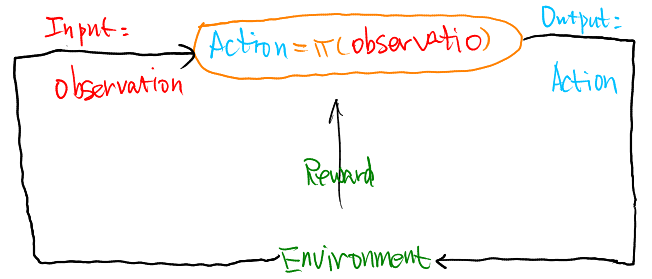• Input: Agent观测到的Observation。
• Output: Agent要采取的Action。通常假设Actor是Stochastic的,也就是说Policy的输出是一个几率。 用Gang的话来说，Policy的输出是一个概率分布

1. Actor和Policy是一个东西。
2. 与Stochastic对应的是Deterministic。想想猜拳游戏, 若是使用Deterministic的策略,很容易被识破。

### # 如何学习一个Actor?

#### # 1. 定义一系列的函数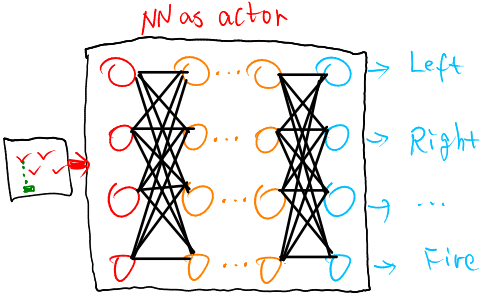• 相比传统的Lookup Table, 玩电玩就不行了，因为状态无限多, 所以就要使用神经网络。
• 神经网络可以举一反三，因为给一个输入(一个画面), 总是可以得到一个输出。神经网络有泛化的作用。即使没有看过一些画面，也有可能会有很好的结果。

#### # 2. 决定函数的好坏

• 给定一个策略$\pi_\theta{(s)}$,这里的$\theta$表示的是神经网络的参数。
• 使用这个策略$\pi_\theta{(s)}$去玩游戏:
• 开始是的观测$s_1$
• 机器决定做出动作$a_1$
• 机器得到奖励$r_1$
• 机器看到$s_2$
• 机器决定做出动作$a_2$
• 机器得到奖励$r_2$
• 机器看到$s_3$
• ...
• 机器决定做出动作$a_T$
• 机器得到奖励$r_T$
• 算出在这一个Episode中得到的:Total reward: $R_{\theta}=\sum_{t=1}^{T}r_t$

• Actor若是Stochastic,即动作本来就是一种可能性。
• 游戏本身也有随机性的,采取同样的Action,每次看到的Observation也是不同的。 也就是说，$R_{\theta}$就是一个随机变量。因此我们的目标就应该变成: 学习去最大化$R_{\theta}$的期望值 $\bar{R}_{\theta}$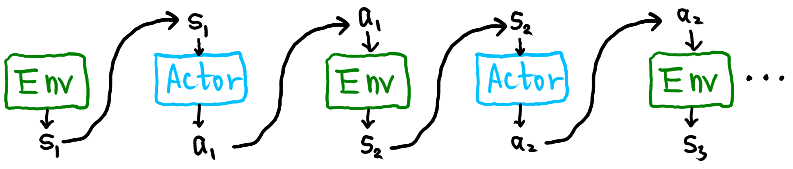• $\tau = {s_1,a_1,r_1,s_2,a_2,r_2,...,s_T,a_T,r_T}$
• $R_{\tau} = \sum_{n=1}^Nr_n$
• 如果你使用一个actor去玩游戏，每一个$\tau$的出现都会有一个概率,它的值取决于actor的参数$\theta$，记为$P(\tau|\theta)$

$\tau$代表了一个游戏过程,具体下来的$\tau$往往有千千万万种, 选择使用某一个Actor去玩这个游戏的时候，可能只会看到其中的某一些$\tau$。有些过程比较容易出现，有些玩很多次都不会遇到(就像是很多人都发现不了游戏中的彩蛋)。因此就出现了$P(\tau|\theta)$

$\bar{R}_{\theta} = E_{\tau \sim p_{\theta}(\tau)}\left[R(\tau) \right] =\sum_{\tau}R(\tau)P(\tau|\theta)$

$\bar{R}_{\theta} = \sum_{\tau}R(\tau)P(\tau|\theta) \approx \frac{1}{N}\sum_{n=1}^{N}R(\tau^{n})$

$\sum_{\tau}P(\tau|\theta) \approx \frac{1}{N}\sum_{n=1}^{N}$

#### # 3. 挑选最好的函数

• 数学语言来描述:
• $\theta^{*} = arg \max \limits_{\theta}\bar{R}_\theta$
• $\bar{R}_{\theta} = \sum_{\tau}R(\tau)P(\tau|\theta)$
• $\theta^{0}$开始
• $\theta^{1} \leftarrow \theta^{0} + \eta \nabla \bar{R}_{\theta^0}$
• $\theta^{2} \leftarrow \theta^{1} + \eta \nabla \bar{R}_{\theta^1}$
• ...

$\bar{R}_{\theta} = \sum_{\tau}R(\tau)P(\tau|\theta)$

$\nabla \overline{R}_{\theta}=\sum_{\tau} R(\tau) \nabla P(\tau | \theta) = \sum_{\tau} R(\tau) P(\tau | \theta) \frac{\nabla P(\tau | \theta)}{P(\tau | \theta)}$

$R_{\tau}$不做微分，可以是一个黑盒。其实大多数情况也确实是一个黑盒子，我们基本不可能完全去理解环境。$R_{\tau}$是环境给我们的。

$=\sum_{\tau} R(\tau) P(\tau | \theta) \nabla \log P(\tau | \theta) = E_{\tau \sim p(\tau|\theta)}\left[R(\tau) \nabla \log p(\tau|\theta)\right]$

$\approx \frac{1}{N} \sum_{n=1}^{N} R\left(\tau^{n}\right) \nabla \log P\left(\tau^{n} | \theta\right)$

$\tau=\left\{s_{1}, a_{1}, r_{1}, s_{2}, a_{2}, r_{2}, \cdots, s_{T}, a_{T}, r_{T}\right\}$

$P(\tau | \theta)= p\left(s_{1}\right) p\left(a_{1} | s_{1}, \theta\right) p\left(r_{1}, s_{2} | s_{1}, a_{1}\right) p\left(a_{2} | s_{2}, \theta\right) p\left(r_{2}, s_{3} | s_{2}, a_{2}\right) \cdots$

$=p\left(s_{1}\right) \prod_{t=1}^{T} p\left(a_{t} | s_{t}, \theta\right) p\left(r_{t}, s_{t+1} | s_{t}, a_{t}\right)$

$P_{\theta}(\tau)=p\left(s_{1}\right) \prod_{t=1}^{T} p_{\theta}\left(a_{t} | s_{t}\right) p\left(s_{t+1} | s_{t}, a_{t}\right)$

$\log P(\tau | \theta) = \log p\left(s_{1}\right)+\sum_{t=1}^{T} \log p\left(a_{t} | s_{t}, \theta\right)+\log p\left(r_{t}, s_{t+1} | s_{t}, a_{t}\right)$

$\nabla \log P(\tau | \theta)=\sum_{t=1}^{T} \nabla \log p\left(a_{t} | s_{t}, \theta\right)$

$\theta^{n e w} \leftarrow \theta^{o l d}+\eta \nabla \overline{R}_{\theta^{o l d}}$

$\nabla \overline{R}_{\theta} \approx \frac{1}{N} \sum_{n=1}^{N} R\left(\tau^{n}\right) \nabla \log P\left(\tau^{n} | \theta\right)=\frac{1}{N} \sum_{n=1}^{N} R\left(\tau^{n}\right) \sum_{t=1}^{T_{n}} \nabla \log p\left(a_{t}^{n} | s_{t}^{n}, \theta\right)$

$= \frac{1}{N} \sum_{n=1}^{N} \sum_{t=1}^{T_{n}} R\left(\tau^{n}\right) \nabla \log p\left(a_{t}^{n} | s_{t}^{n}, \theta\right)$

• $R(\tau^n)$为正, 那么我们就调整$\theta$来增大$p(a_t^n|s_t^n)$
• $R(\tau^n)$为负, 那么我们就调整$\theta$来减小$p(a_t^n|s_t^n)$

Step 1: 收集数据 给定一个策略$\pi_{\theta}$, 让Agent去玩N次游戏，收集下面一组数据: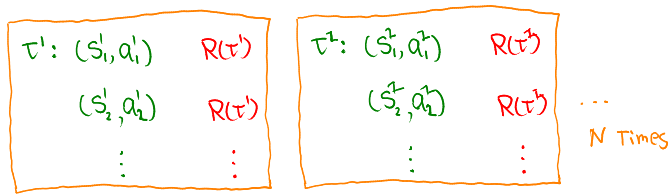Step 2: 更新网络参数 这里的N组数据就相当于是一组Mini-Batch, 因此是N次游戏之后，利用:

$\nabla \overline{R}_{\theta}= \frac{1}{N} \sum_{n=1}^{N} \sum_{t=1}^{T_{n}} R\left(\tau^{n}\right) \nabla \log p_{\theta}\left(a_{t}^{n} | s_{t}^{n}\right)$

$\nabla \log p(a_t^n|s_t^n,\theta) = \frac{\nabla p\left(a_{t}^{n} | s_{t}^{n}, \theta\right)}{p\left(a_{t}^{n} | s_{t}^{n}, \theta\right)}$

$\tau^{13}$, 采取action $a$ $R(\tau^{13}) =2$
$\tau^{15}$, 采取action $b$ $R(\tau^{15}) =1$
$\tau^{17}$, 采取action $b$ $R(\tau^{17}) =1$
$\tau^{33}$, 采取action $b$ $R(\tau^{33}) =1$

$\nabla \overline{R}_{\theta} \approx \frac{1}{N} \sum_{n=1}^{N} \sum_{t=1}^{T_{n}} \left[R\left(\tau^{n}\right)-b\right] \nabla \log p\left(a_{t}^{n} | s_{t}^{n}, \theta\right)$

$\theta=\left\{w_{1}, w_{2}, \cdots, b_{1}, \cdots\right\}$

$\nabla \overline{R}_{\theta}=\left[\begin{array}{c}{\partial \overline{R}_{\theta} / \partial w_{1}} \\ {\partial \overline{R}_{\theta} / \partial w_{2}} \\ {\vdots} \\ {\partial \overline{R}_{\theta} / \partial b_{1}} \\ {\vdots}\end{array}\right]$

$\frac{d\ log(f(x))}{d x}=\frac{1}{f(x)} \frac{d f(x)}{d x}$

$\nabla f(x)= f(x) \nabla \log f(x)$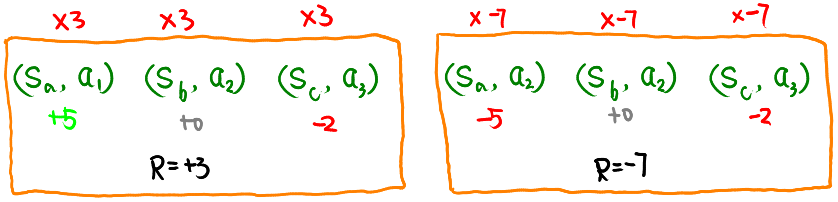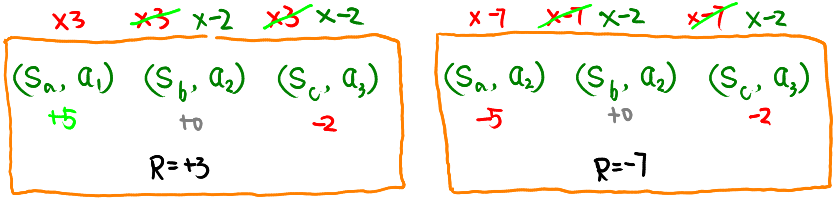$R(\tau^n) \rightarrow \sum_{t^{\prime}=t}^{T_{n}} r_{t^{\prime}}^{n} \rightarrow \sum_{t^{\prime}=t}^{T_{n}} \gamma^{t^{\prime}-t} r_{t^{\prime}}^{n}$